# Undetermined Coefficients, Method of

The following article is from The Great Soviet Encyclopedia (1979). It might be outdated or ideologically biased.

## Undetermined Coefficients, Method of

a method used in mathematics for finding the coefficients of expressions whose form is previously known. For example, the fraction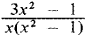can be represented on the basis of theoretical considerations in the form of the sum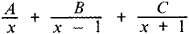where A, B, and C are the coefficients to be determined. To find the coefficients, we first equate the second expression to the first: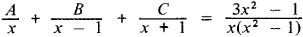Then, clearing fractions and collecting terms on the left with like powers of x, we obtain

(A + B + C)x2 + (B - C) - A ═ 3x2 - 1

Since the last equation must be valid for all values of x, the coefficients with like powers of x on the right and left must be identical. Thus, three equations are obtained to determine the three unknowns, namely, A + B + C ═ 3, B - C ═ 0, and A ═ 1 from which ABC ═ 1. Consequently,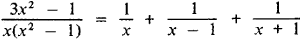The validity of this equation is easily verified directly.

Suppose that it is also necessary to represent the fraction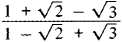in the form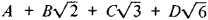where A, B, C, and D are unknown rational coefficients. We equate the second expression to the first: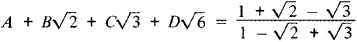or, clearing fractions, taking out where possible the rational factors from under the square root sign, and reducing like terms on the left side, we obtain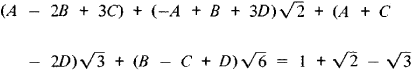Such an equality is possible only if the rational terms on both sides are equal and if the coefficients of the same radicals are equal. Thus, four equations are obtained for finding the unknown coefficients A, B, C, and D, namely, A, -2B + 3C ═ 1, -A + B + 3D ═ 1, A + C - 2D ═ -1 and B - C + D ═ 0, from which A ═ 0, B ═ -1/2, C ═ 0, and D ═ 1/2, that is,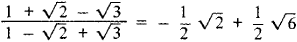In these examples, the success of the method of undetermined coefficients depends on the correct choice of the expressions whose coefficients are to be found. If in the last example the expression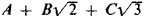was taken instead of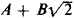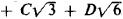, then we would obtain for the three coefficients A, B, and C, reasoning as before, the four equations A - 2B + 3C ═ 1, A + C ═ -1, and B - C ═ 0 which cannot be satisfied for any choice of numbers A, B, and C.

Applications of the method of undetermined coefficients to problems in which the number of unknown coefficients is infinite are of particular importance. Such problems include the division of power series and the determination of the solution of a differential equation in the form of a power series. For example, suppose we want to find the solution of the differential equation y ” + xy ═ 0 such that y ═ 0 and y1 ═ 1 when x ═ 0. It follows from the theory of differential equations that such a solution exists and has the form of a power series

y ═ x + c2x2 + c3x3 + c4 x4 + c5x5 + . . .

Substituting this expression for y and the expression

2c2 + 3 . 2c3x + 4 . 3c4x2 + 5·4c5x3 + . . .

for y “ in the equation y” + xy ═ 0, then multiplying by x and collecting terms with like powers of x, we obtain

2c2 + 3·2c3x + (1 + 4·3c4) x2

+ (c2 + 5·4c5)x3 + . . . ═ 0

from which in determining the unknown coefficients we obtain an infinite system of equations, namely, 2c2 ═ 0, 3.2C3 ═ 0, 1 + 4.3c4 ═ 0, c2 + 5.4C5 ═ 0.....

Successively solving these equations, we obtain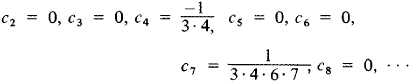that is,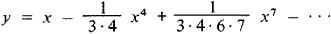### REFERENCES

Smirnov, V. I. Kurs vysshei matematiki, vol. 1, 23rd ed., Moscow, 1974; vol. 2, 20th ed., Moscow, 1967.
Stepanov, V. V. Kurs differential’nykh uravnenii, 8th ed. Moscow, 1959.